# Dungeon generation using BSP trees

Essentially, a binary space partition algorithm will recursively divide a surface into two for a number of iterations. A binary tree provides the most obvious data structure to traverse the different levels of partitions.

For partitioning, in each iteration the direction of the division -horizontal or vertical- is assigned randomly and so is the size of the first partition, while the second just takes the remaining of the space. Later, on the leafs of the tree (down-most nodes), rooms can be grown within the container, be it randomly or by assigning some constraints that suit the generation. Finally, by connecting the center of each one of the partitions with his brother, all the partitions get connected and accessible.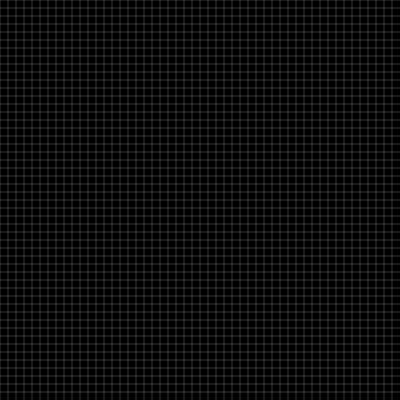Maybe it’s a good idea to check the interactive demo of the code that comes ahead before starting. To increase the number of iterations make sure to increase the map size accordingly.

Let’s get into it. First we need a toy implementation of a binary tree. Do not consider the following feature proof or efficient code-wise.

```var Tree = function( leaf ) {
this.leaf = leaf
this.lchild = undefined
this.rchild = undefined
}```

That’s it. Well, we might sooner or later need some auxiliary functions to get an specific level of the tree and to get the down-most bottom leafs of it, be it for debugging or just for the exercise.

```Tree.prototype.getLeafs = function() {
if (this.lchild === undefined && this.rchild === undefined)
return [this.leaf]
else
return [].concat(this.lchild.getLeafs(), this.rchild.getLeafs())
}

Tree.prototype.getLevel = function(level, queue) {
if (queue === undefined)
queue = []
if (level == 1) {
queue.push(this)
} else {
if (this.lchild !== undefined)
this.lchild.getLevel(level-1, queue)
if (this.rchild !== undefined)
this.rchild.getLevel(level-1, queue)
}
return queue
}

Tree.prototype.paint = function(c) {
this.leaf.paint(c)
if (this.lchild !== undefined)
this.lchild.paint(c)
if (this.rchild !== undefined)
this.rchild.paint(c)
}```

This should be enough to keep things forward. Again, an array mapped tree would avoid us some unnecessary recursive steps, but this is a demo, right?

Now, some utils that will come handy

```var Point = function(x, y) {
this.x = x
this.y = y
}

function random(min, max) {
return Math.floor(Math.random() * (max - min + 1) + min)
}```

Next thing we need a container prototype. Easy as pie:

```var Container = function(x, y, w, h) {
this.x = x
this.y = y
this.w = w
this.h = h
this.center = new Point(
this.x + (this.w/2),
this.y + (this.h/2)
)
}

Container.prototype.paint = function(c) {
c.strokeStyle = "#0F0"
c.lineWidth   = 2
c.strokeRect(this.x * SQUARE, this.y * SQUARE,
this.w * SQUARE, this.h * SQUARE)
}```

Okay, let’s build this tree. We need a function that grows a binary tree of containers.

```function split_container(container, iter) {
var root = new Tree(container)
if (iter != 0) {
var sr = random_split(container)
root.lchild = split_container(sr, iter-1)
root.rchild = split_container(sr, iter-1)
}
return root
}```

And now the actual function that splits a container

```function random_split(container) {
var r1, r2
if (random(0, 1) == 0) {
// Vertical
r1 = new Container(
container.x, container.y,             // r1.x, r1.y
random(1, container.w), container.h   // r1.w, r1.h
)
r2 = new Container(
container.x + r1.w, container.y,      // r2.x, r2.y
container.w - r1.w, container.h       // r2.w, r2.h
)
} else {
// Horizontal
r1 = new Container(
container.x, container.y,             // r1.x, r1.y
container.w, random(1, container.h)   // r1.w, r1.h
)
r2 = new Container(
container.x, container.y + r1.h,      // r2.x, r2.y
container.w, container.h - r1.h       // r2.w, r2.h
)
}
return [r1, r2]
}```

Good, now let’s throw it into a canvas! The wording here might look a bit weird, just assume we are entering this in a development console.

```var canvas       = document.getElementById('viewport')
var MAP_SIZE     = 50
var SQUARE       = canvas.width / MAP_SIZE
var N_ITERATIONS = 4

var c_context = canvas.getContext('2d')

var main_container = new Container(0, 0, canvas.width, canvas.height)
var container_tree = split_container(main_container, N_ITERATIONS)

c_context.fillStyle = "#000"
c_context.fillRect(0, 0, canvas.width, canvas.height)
container_tree.paint(c_context)```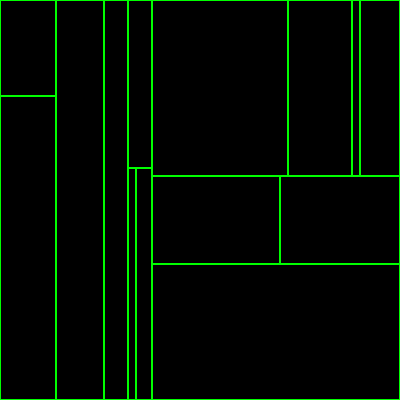Woosh, not much impressive, this barely resembles a Piet Mondrian scam.

### The ugly bits

Randomness it’s all good and fun, until you find out that containers sized too small or too large will result in weird looking results, so here I am discarding any container with a horizontal or vertical ratio smaller than a predefined one. Too aggressive ratios will follow with growing the stack size out of the heap limit.

I do not know if this is the best solution, or if I should not worry about too small partitions, or for instance if I could generate the random width or height with a minimum value (though, I do not like that, as it makes the algorithm code as straightforward. If we define a minimum of 3 squares, what happens when you are confronted by an hypothetical partition of 4 squares?).

```function random_split(container) {
var r1, r2
if (random(0, 1) == 0) {
// Vertical
r1 = new Container(
container.x, container.y,             // r1.x, r1.y
random(1, container.w), container.h   // r1.w, r1.h
)
r2 = new Container(
container.x + r1.w, container.y,      // r2.x, r2.y
container.w - r1.w, container.h       // r2.w, r2.h
)

var r1_w_ratio = r1.w / r1.h
var r2_w_ratio = r2.w / r2.h
if (r1_w_ratio < W_RATIO || r2_w_ratio < W_RATIO) {
return random_split(container)
}
}
} else {
// Horizontal
r1 = new Container(
container.x, container.y,             // r1.x, r1.y
container.w, random(1, container.h)   // r1.w, r1.h
)
r2 = new Container(
container.x, container.y + r1.h,      // r2.x, r2.y
container.w, container.h - r1.h       // r2.w, r2.h
)

var r1_h_ratio = r1.h / r1.w
var r2_h_ratio = r2.h / r2.w
if (r1_h_ratio < H_RATIO || r2_h_ratio < H_RATIO) {
return random_split(container)
}
}
}
return [r1, r2]
}```

Ok, run again!

```var canvas           = document.getElementById('viewport')
var MAP_SIZE         = 50
var SQUARE           = canvas.width / MAP_SIZE
var N_ITERATIONS     = 4
var H_RATIO          = 0.45
var W_RATIO          = 0.45

var c_context = canvas.getContext('2d')

var main_container = new Container(0, 0, canvas.width, canvas.height)
var container_tree = split_container(main_container, N_ITERATIONS)

c_context.fillStyle = "#000"
c_context.fillRect(0, 0, canvas.width, canvas.height)
container_tree.paint(c_context)```Much more pleasing to the eye. It’s true that a pattern-like structure can be seen and by discarding small results epic randomness is also being discarded, with the possibility to delete rooms that are too small or impossible (hence, resulting in one less room in the map). In any case, the option of discarding is left open, and the results wielded are much more intelligible just for this post.

At the start I was looking after a city-like partition. To see if BSP will yield me what I was looking after, I did set the recursion level to 9 with a map size of 500 squares. The results resemble what I want, and give me enough material to move to the next stage.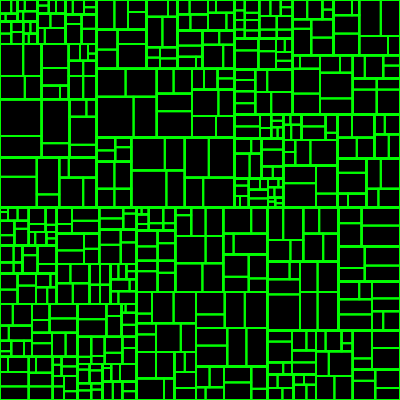### Into the rooms and paths

For the purpose of my generator both rooms and paths are a bit out of the scope as it is not exactly what I am looking for yet. However, I will include them for the sake of completeness, so inaccuracies should be expected.

As mentioned earlier in the post, when we are happy with a recursion level, rooms are placed within each container. The sizing on the room can be defined by any rules. In this example, rooms are grown with a random padding ranging from 0 to a third of the room size (for each of the sides). By allowing it to be 0, the possibility of touching rooms is contemplated, which should give interesting results.

```var Room = function( container ) {
this.x = container.x + random(0, Math.floor(container.w/3))
this.y = container.y + random(0, Math.floor(container.h/3))
this.w = container.w - (this.x - container.x)
this.h = container.h - (this.y - container.y)
this.w -= random(0, this.w/3)
this.h -= random(0, this.w/3)
return this
}
Room.prototype.paint = function(c) {
c.fillStyle = "#888"
c.fillRect(this.x * SQUARE, this.y * SQUARE,
this.w * SQUARE, this.h * SQUARE)
}```

Using the helper function to get the leafs of the tree rooms are created dependent of each one of them and later its paint function is called.

```var canvas           = document.getElementById('viewport')
var MAP_SIZE         = 50
var SQUARE           = canvas.width / MAP_SIZE
var N_ITERATIONS     = 4
var H_RATIO          = 0.45
var W_RATIO          = 0.45

var c_context = canvas.getContext('2d')

var main_container = new Container(0, 0, canvas.width, canvas.height)
var container_tree = split_container(main_container, N_ITERATIONS)

c_context.fillStyle = "#000"
c_context.fillRect(0, 0, canvas.width, canvas.height)
container_tree.paint(c_context)
var leafs = container_tree.getLeafs()
for (var i = 0; i < leafs.length; i++) {
new Room(leafs[i]).paint(c_context)
}```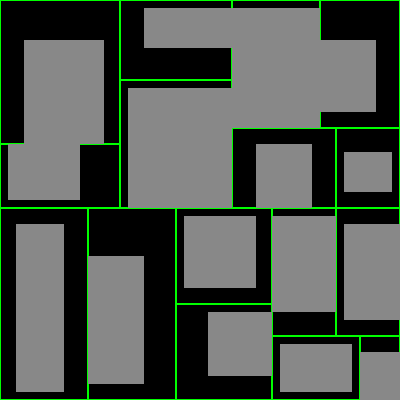Sweet. Now let’s add a way for paths to be drawn on the canvas. Again, the purpose of this demo is just on drawing the stuff, so we are not going to save them in any structure, nor carve it into a tile map. Let’s see what happens if we draw a line between the centers of containers with the same parent.

```Container.prototype.drawPath = function(ctx, container) {
ctx.beginPath()
ctx.lineWidth = SQUARE
c.strokeStyle = "#888"
c.moveTo(this.center.x * SQUARE, this.center.y * SQUARE)
c.lineTo(container.center.x * SQUARE, container.center.y * SQUARE)
c.stroke()
}
var draw_paths = function(ctx, tree) {
if (tree.lchild == undefined || tree.rchild == undefined)
return
tree.lchild.leaf.drawPath(ctx, tree.rchild.leaf)
draw_paths(ctx, tree.lchild)
draw_paths(ctx, tree.rchild)
}

var canvas           = document.getElementById('viewport')
var MAP_SIZE         = 50
var SQUARE           = canvas.width / MAP_SIZE
var N_ITERATIONS     = 4
var H_RATIO          = 0.45
var W_RATIO          = 0.45

var c_context = canvas.getContext('2d')

var main_container = new Container(0, 0, canvas.width, canvas.height)
var container_tree = split_container(main_container, N_ITERATIONS)

c_context.fillStyle = "#000"
c_context.fillRect(0, 0, canvas.width, canvas.height)
container_tree.paint(c_context)
draw_paths(c_context, container_tree)
var leafs = container_tree.getLeafs()
for (var i = 0; i < leafs.length; i++) {
new Room(leafs[i]).paint(c_context)
}```Good as done. Now, what about some cleaning up?

```var Map = function(canvas) {
this.width  = canvas.width
this.height = canvas.height
this.ctx    = canvas.getContext('2d')
this.c_tree = undefined
this.rooms  = []
}

Map.prototype.init = function() {
var m_container = new Container(0, 0, MAP_SIZE, MAP_SIZE)
this.c_tree = split_room(m_container, N_ITERATIONS)
this.growRooms()
}

Map.prototype.growRooms = function() {
var leafs = this.c_tree.getLeafs()
for (var i = 0; i < leafs.length; i++)
this.rooms.push(new Room(leafs[i]))
}

Map.prototype.clear = function() {
this.ctx.fillStyle = "#000"
this.ctx.fillRect(0, 0, this.width, this.height)
}

Map.prototype.drawGrid = function() {
this.ctx.beginPath()
this.ctx.strokeStyle = "rgba(255,255,255,0.4)"
this.ctx.lineWidth = 0.5
for (var i = 0; i < MAP_SIZE; i++) {
this.ctx.moveTo(i * SQUARE, 0)
this.ctx.lineTo(i * SQUARE, MAP_SIZE * SQUARE)
this.ctx.moveTo(0, i * SQUARE)
this.ctx.lineTo(MAP_SIZE * SQUARE, i * SQUARE)
}
this.ctx.stroke()
}

Map.prototype.drawContainers = function() {
this.c_tree.paint(this.ctx)
}

Map.prototype.drawRooms = function() {
for (var i = 0; i < this.rooms.length; i++)
this.rooms[i].paint(this.ctx)
}

Map.prototype.drawPaths = function(tree) {
if (tree.lchild == undefined || tree.rchild == undefined)
return
tree.lchild.leaf.drawPath(this.ctx, tree.rchild.leaf)
this.draw_paths(tree.lchild)
this.draw_paths(tree.rchild)
}

Map.prototype.paint = function() {
this.clear()
this.drawGrid()
this.drawContainers()
this.drawRooms()
this.drawPaths()
}```

Which now can be run as

```var map = new Map(document.getElementById('viewport'))
map.init()
map.paint()```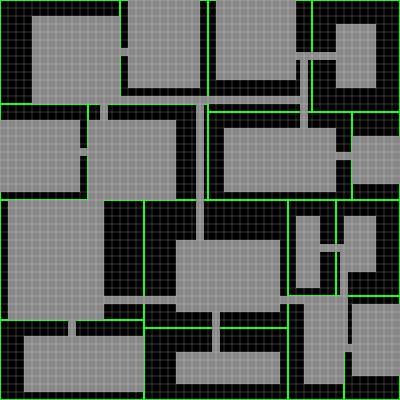Feel free to check the playground demo at /demos/dungeon.

## 10 thoughts on “Dungeon generation using BSP trees”

1.apol says:

Dungeons!! :O

1.eskerda says:

:D

2.LuisQuin says:

Maybe is there a little problem in your …?
Container.prototype.paint = function(c) {
c.strokeStyle = “#0F0”
c.lineWidth = 2
c.strokeRect(this.x * SQUARE, this.y * SQUARE,
this.w * SQUARE, this.h * SQUARE)
}
Shouldn’t it be …?
c.strokeRect(this.x, this.y,
this.w * SQUARE, this.h * SQUARE)

3.fnaith says:

Dungeons! ヾ(●゜▽゜●)

This site uses Akismet to reduce spam. Learn how your comment data is processed.